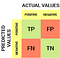# Performance Evaluation Metrics

Evaluating a machine learning model is as much important as building it, if not more. The only way by which we can know that our model draws out accurate conclusions is by evaluating its performance by passing it through a number of criterion and analyzing the results obtained.

This article aims at providing the readers, a brief idea about the various performance evaluation metrics used. However, before moving on to the main article, let’s have a look at the basic terms and definitions that you’ll need to know so that you can understand this article better.

A true positive is an outcome where the model correctly predicts the positive class. Example: A COVID-19 positive person is correctly predicted to be positive.

A true negative is an outcome where the model correctly predicts the negative class. Example: A COVID-19 negative person is correctly predicted to be negative.

A false positive is an outcome where the model incorrectly predicts the positive class. Example: A COVID-19 negative person is incorrectly predicted to be positive.

A false negative is an outcome where the model incorrectly predicts the negative class. Example: A COVID-19 positive person is incorrectly predicted to be negative.

# Classification Accuracy

In terms of the terms we discussed above, accuracy can be described as:

Classification accuracy often leads to misleading results, especially in the case of unbalanced classes, which is when we have different number of samples in different classes.

# Confusion MatrixConfusion Matrix

# Precision

If we make the precision as high as possible, i.e., almost equal to 1, the recall of our model would decrease because of the high number of false negatives. For some machine learning models, we need both precision and recall to be balanced with each other. For such a scenario, we calculate another metric called F1 Score.

# F1-Score

F1-Score= 2*(Recall * Precision) / (Recall + Precision)

# False Positive Rate

This was all about the basics of the various performance evaluation metrics mainly used in classification. I hope I was able to clear up the basics!

You can find me on LinkedIn at: https://www.linkedin.com/in/manasvita-sharma-b35315171/

Electronics and communications engineering student | Avid reader

## More from Manasvita Sharma

Electronics and communications engineering student | Avid reader